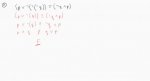# Truth tables help: "p or not-(not-(not-q))) = not-q and p" ?

#### Phatfoo

##### New member
Hello all. I am new here and will introduce myself in the appropriate forum.

I am first year CompSci and maths is a large part of my course. I was terrible at school maths, but I am enjoying varsity maths, albeit a struggle. Which is why I'm here! I have been doing exercises and would like your help.

The question here is "Is this true or false?"If my answer is incorrect, then would you so kind as to show me where I went wrong?

#### ksdhart2

##### Full Member
It looks like the problem statement is written in black, and your work is in red. So the problem statement is:

$$\displaystyle \left(P \vee \neg(\neg(\neg Q)) \right) \equiv \left( \neg Q \wedge P \right)$$

Assuming this is the case, your answer is correct, although your justification is wrong. On the left-hand side, two of the negations cancel out and then some parentheses collapse. Everything you did is fine up until this step:

$$\displaystyle \left( P \vee \neg Q \right) \equiv \left(\neg Q \wedge P \right)$$

Your final step is incorrect because:

$$\displaystyle \left( P \vee \neg Q \right) \not\equiv \left( P \wedge Q \right)$$

We can easily see this equivalence does not hold by testing some values. Suppose P is true and Q is false. Then we'd have:

$$\displaystyle \left( \text{TRUE} \vee \neg \text{FALSE} \right) \stackrel{?}{\equiv} \left( \text{TRUE} \wedge \text{FALSE} \right)$$

$$\displaystyle \left( \text{TRUE} \vee \text{TRUE} \right) \stackrel{?}{\equiv} \text{FALSE}$$

$$\displaystyle \text{TRUE} \not\equiv \text{FALSE}$$

Similarly, we can see the equivalence used on the right-hand side doesn't hold either. Suppose P and Q are both true:

$$\displaystyle \left(\neg Q \wedge P \right) \not\equiv \left(Q \vee P \right)$$

$$\displaystyle \left(\neg \text{TRUE} \wedge \text{TRUE} \right) \stackrel{?}{\equiv} \left(\text{TRUE} \vee \text{TRUE} \right)$$

$$\displaystyle \left(\text{FALSE} \wedge \text{TRUE} \right) \stackrel{?}{\equiv} \text{TRUE}$$

$$\displaystyle \text{FALSE} \not\equiv \left(\text{TRUE} \right)$$

Instead, starting again from the last known good line, we can swap the order of P and Q and make the statement:

$$\displaystyle \left( \neg Q \vee P \right) \equiv \left(\neg Q \wedge P \right)$$

And from there, it should be obvious to see why the two expressions cannot possibly be equivalent.

#### Phatfoo

##### New member
Thank you so much! That really helped me understand much better!

I see now what I did wrong.